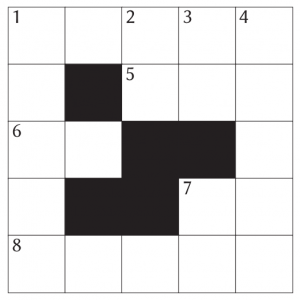# Chalkdust issue 11 puzzle hunt #4

Humbug sets the fourth puzzle. Can you solve it?The day is finally here: issue 11 is out now! To help you celebrate launch day in style, we’ve set a puzzle hunt: throughout the day, we are posting a series of puzzles. The answers to these puzzles form clues to the four-digit code for the door to let you into the Chalkdust issue 11 secret backstage lounge.

The fourth puzzle is set by Humbug. You can see the puzzle below, or download it as a pdf.

Be sure to come back at 5pm when the fifth puzzle will be posted.

## Humbug’s puzzle

Although many of the clues have multiple answers, there is only one solution to the completed crossnumber. As usual, no numbers begin with 0.

The number of copies of the digit 1 in the completed crossnumber is one more than a digit of the code.#### Across

• 1 The sum of this number’s digits is 12. (5)
• 5 The middle digit of this number is equal to the first digit of 4D. (3)
• 6 The product of the digits of 5A. (2)
• 7 The sum of this number’s digits is 7. (2)
• 8 1A reversed. (5)

#### Down

• 1 The sum of this number’s digits is 14. (5)
• 2 This number is equal to 2 multiplied by the sum of its digits. (2)
• 3 This number is equal to 3 multiplied by the sum of its digits. (2)
• 4 1D reversed. (5)
• 7 The product of this number’s digits is 7. (2)